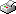Print This Page Boris Lorenc -- doctoral thesis -- Two Topics in Survey Methodology Abstract Modelling the response process in establishment surveys proceeded thus far by adding steps of operational character to the core cognitive steps of the individual cognitive model. Here (Paper 1) a larger unit of the cognitive analysis is posited: that part of the enterprise that is engaged in responding to a survey, consisting of one or more employees, the tools they use and the established practices of record keeping and survey responding. Propagation of representational states is the key concept used for analysing the response process. A pilot study conducted on a survey of schools (Paper 2) illustrates the ability of the approach to identify and suggest adjustment of items of questionable accuracy and addresses the possibility of quantifying accuracy of collected data. In order to assess the skewness of a nonprobability web sample in relation to the targeted population, George Terhanian proposed a parallel availability of a probability sample from the population and the use of the assessment to reweight data in the nonprobability sample. The parallel availability of the two samples constitutes the double samples setup. Propensity scores have been suggested as a way of adjusting web samples. Here (Paper 3) the propensity score approach is broadened by (i ) putting it in a wider context of the double samples setup applicable in other .elds as well, (ii ) showing the theoretical unbiasedness of the estimator and (iii ) specifying the assumptions that need to hold. In a simulation, the effects of the factors where an analytic approach was difficult or impossible to apply were investigated. The paper also contains a simple example of applying the technique, with a step-by-step "how to". Inference in the double samples setup proceeded thus far by modelling either the probabilities of inclusion in the nonprobability sample or the study variable. A more general framework is presented (Paper 4) that models both components at the same time. From the model an estimator is derived. In a simulation, it is compared with three estimators that model just one of the two components. Finally, a nonparametric estimator for the situation of double samples is presented (Paper 5). Here, the values of the study variable. missing by design in the probability sample. are imputed according to a distance measure on auxiliary information. The mean of a number of closest units is imputed with the aim of reducing the variance of the estimator. For the case of univariate auxiliary information treated in the study, an expression for the variance and a variance estimator for this estimator is also presented and veri.ed in a simulation. Keywords: response process, establishment surveys, socially distributed cognition, response burden, measurement error, nonprobability samples, web surveys, double samples, propensity scores, model-based estimation, imputation, nonparametric imputation, weighting. ISBN 91-7155-253-7